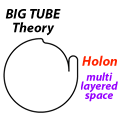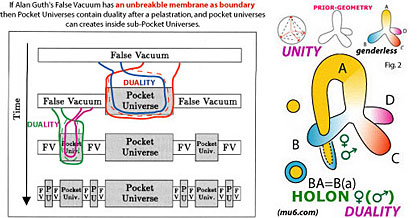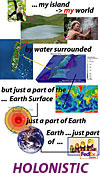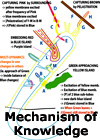SPACETIME

 As an introduction I just want to point first to Alan Guth's reference to a false vacuum and the creation of POCKET UNIVERSES. As you will notice in below image such idea is conceptual very identical if the boundary of such general false vacuum is considered to be non-breakable. You will notice that Alan Guth's pocket universes are separated by a false vacuum, in our idea this is the isolation that follows from a pelatration. Pocket Universes are then holons. So particles are pocket universes too.In our approach Spacetime is a dynamic membrane, ruled by kinetics. As you know Einstein looked for a pure kinetic approach.

Next you can find the principles of pelastration explained in just kinetic terms.
There are an absolute minimum of actors.

The absolute minimum concept is this one.

1. There is completely closed system (CS) with only it's own boundary. (1 postulate)
2. Nature shows us there is movement (GKE : General Kinetic Energy). (observation)
3. The dynamic system (DS) has a General Elastic Boundary (GEB). (conclusion)
4. The moving system acts like a perpetual mobile, since (1) there are no losses.(conclusion)
5. All interactions and self-sustaining mechanisms must thus be generated inside the system.(conclusion)
6. Interactions imply that there must be structural dualism (unbalance) embedded in the system.
7. Dualism in the system is only possible if 'isolation' is available.
8. Since there is only boundary available, the boundary itself must be acting as isolator.
9. Thus the system must contain isolated zones created by a doubling mechanism of the boundary.
10. Isolated zones must contain several layers of boundary, thus restructured boundary.
11. Isolated zones have a structure.
12. GKE changes the inter-position of the boundaries (joined boundary) inside the isolated zones.
13. In isolated zones the boundaries have interacting GKE-properties, resulting in specific LKE (Local Kinetic Energy).
14. GKE changes constantly the position of the isolated zones in the dynamic system.
15. GKE changes constantly the position of the boundaries in each isolated zone (IZ), thus it's LKE.
16. Via it's boundaries the LKE of each isolated zone feeds back on the GKE.
17. Isolated zones and their LKE influence the spatial properties of the GEB (General Elastic Boundary).
18. Changes of positions of isolated zones (14) provoke interactions (actions and reactions) between IZ's.
19. Interactions between IZ's provoke changes in their proper LKE, thus in their boundaries.
20. Interactions between IZ's can provoke Combinations or Groups of IZ's (GIZ's), i.e. by knotting, by embedding, etc.
21. GIZ's have specific Group LKE which feeds back on the GKE.
22. IZ's and GIZ's can interact and influence each others boundaries.
23. By GKE all boundaries in IZ's and GIZ's are constantly in movement.
24. The constant movement in IZ's and GIZ's create a number of phenomena described in physics as interacting or independent forces, fields or waves but which are the result of the internal LKE in IZ's and GIZ's.
25. The specific boundaries ( restructured boundary) in IZ's and GIZ's create a number of phenomena observed by physicists as matter, light, radiation, magnetism, particles, density, ... but which as the result of the internal dynamic geometry in IZ's and GIZ's.
26. The constant position changes of IZ's and GIZ's create a number of phenomena described in physics as velocity, acceleration, position, dimensions ... but which are the result of (26.1) the interactions between IZ's and GIZ's, (26.2) GKE, and (26.3.) the interaction of an IZ or GIZ's with the GEB.
27. IZ's being part of the GEB are located on the GEB, but add an additional layer (IZ-layer) on the GEB, and GIZ's add an additional layer on the IZ-layer, which is describes in physics observations as BEC, Casimir effects, monopoles, quantum packages, ... or explained as holographic, implicit order, towers, etc.
28. Since GEB is the base of the IZ's, IZ's are the base of the GIZ's, ... and GIZ's can build to more complex CIZ's their is an hierarchic layering principle with historical non-commutative values.
29. The more IZ-layers are located on a GEB-zone the more the elasticity of this GEB-zone will be influenced, meaning: become less flexible (more rigid), also called: the bending of spacetime.
30. The continuos inter-action between the GEB and IZ's (and GIZ's) is described in physics as gravity, but physicists have the tendency only to account the effect of IZ's (and GIZ's) on GEB (described as spacetime).
31. Phenomena (i.e. mass) and actions (i.e. acceleration) of IZ's cause re-actions in the GEB, like actions of the GEB cause reactions between and in IZ's (and GIZ's).
32. ... more to continue ( i.e. dimensional differences, resonance, etc).

Now an essential question: is g-uv = g-mu,nu ?

The actual interpretation of Einsteins GR is that the gravitational field is equal to the metric tensor, written as g-uv = g-mu,nu. But this is not correct.

This brings us to next:

PG (Prior-Geometry) = GKE (General Kinetic Energy)
PG = g-uv ( gravitational field, but better described as: gravitational brane)
But
g-uv ≠ g-mu,nu (the metric tensor)
Since
g-uv = GKE
g-mu,nu = ∑LKE (totality of Local Kinetic Energy)

We can descibe GKE also as Non-LKE
The total Closed System (CS), our universe, is thus
Non-LKE + ∑LKE = CS

Indeed the framework of the universe only starts when we speak of Local Kinetic Energy. Only when we have local 'discrete' zones/events they can influences each other. Since the IZ's, GIZ's and CIZ's are connected
with and by the dynamic spacetime brane the positions between IZ's is ALWAYS relative and CHANGING.

 [ spacetime 1 ] [ spacetime 2 ] [ spacetime 3 ]